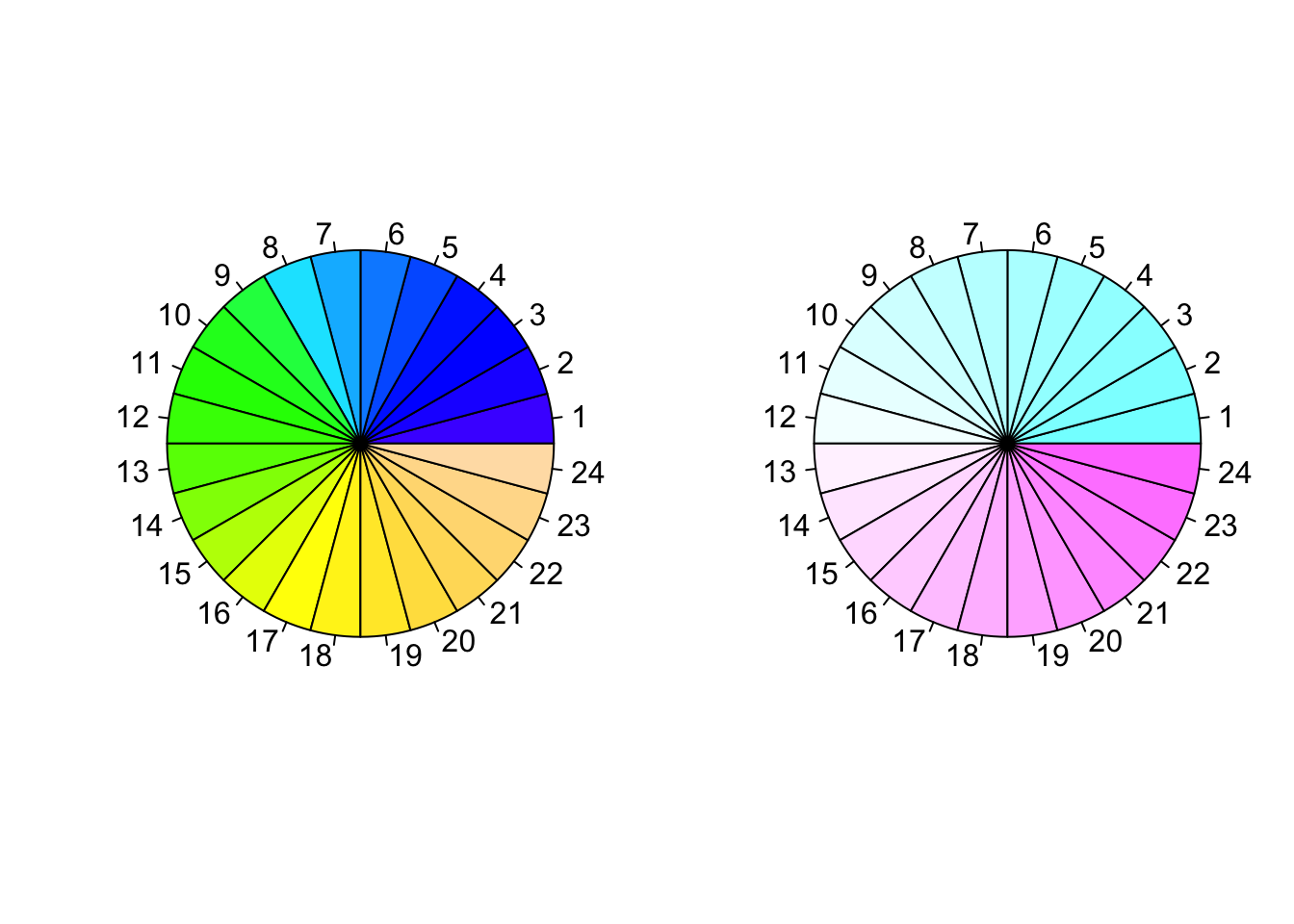# Basic graphics in R

R is a great tool for creating plots, maps and graphics. R includes many built-in graphics functions that would help to draw plots easily. R is a very self explanatory environment in terms of documentation.

You may find more about R programming in:

## Functions

The following is a list of the most practical graphics functions in R:

• plot: Generic function for plotting of R objects
• lines: A generic function taking coordinates and joining the corresponding points with line segments
• ablines: This function adds one or more straight lines through the current plot
• points: A generic function to draw a sequence of points at the specified coordinates
• panel.smooth: Simple panel plot
• smoothScatter: Produce a smoothed color density representation of a scatterplot,
• pairs: Produce a matrix of scatter plots
• hist: Computes a histogram of the given data values
• acf: Computes (and by default plots) estimates of the autocorrelation function
• boxplot: Produce box-and-whisker plot(s) of the given (grouped) values
• barplot: Creates a bar plot with vertical or horizontal bars
• dotchart: Draw a Cleveland dot plot
• pie: Draw a pie chart
• polygon: Polygon draws the polygons whose vertices are given in x and y
• symbols: This function draws symbols on a plot
• arrows: Add arrows to a plot
• axis: Add an axis to a plot
• stars: Draw star (segment) plots and spider (radar) plots
• contour: Create a contour plot, or add contour lines to an existing plot
• image: Creates a grid of colored or gray-scale rectangles with colors corresponding to the values in z
• persp: This function draws perspective plots of a surface over the x–y plane

There are several graphical parameters that can be specified in the graphics functions or through parameter function, par(), before the graphs. Most important graphical parameters:

• ann: Plots annotations which is axis titles and overall titles, ann = FALSE means no titles
• bg: Background color
• bty: Border type, bty = "n" means border type is NULL
• cex: A value giving the amount which plotting text and symbols should be magnified relative to the default
• las: Labels axis style
• lty: Lines types
• lwd: Lines width
• main: Plot main title
• mfrow: Multiple figures in rows, it is a vector of the form c(nr,nc)
• pch: Points character
• xaxt/yaxt: X-axis/y-axis style, xaxt = "n" means no scale on x-axis
• xlab/ylab: X-axis/y-axis label
• xlim/ylim: X-axis/y-axis limit

This tutorial shows how to apply above functions and parameters to draw graphs by R. We will also, briefly learn how to generate publication style tables and maps.

## Scatter plots, lines and points

x = 1:100
y = rnorm(100, 0, 2)

## Left plot
par(mfrow = c(1,2))
plot(x, y, las = 1, xaxt = "n")
axis(1, at = c(0,25,50,75,100), labels = c('O','A','B','C','D'))
panel.smooth(x, y, col = "blue")

## Right plot
plot(x, y, type = "l", col = "blue", ylab = "", yaxt = "n", las = 2)
lines(smooth(y), col = "red", lty = 1, lwd = 1.4)
abline(h = 3, v = 80, col = "orange", lwd = 3)
abline(h = 0, lty = 2, lwd = 2)
points(c(20,40,60,80,100), c(0,-1,1,3,2), col = "black", bg = "green", pch = 21, cex = 2)
text(x = c(40,80), y = c(-1.2,3.2), labels = c("MIN","MAX"), pos = c(1,3), col = "red",  cex = 0.9)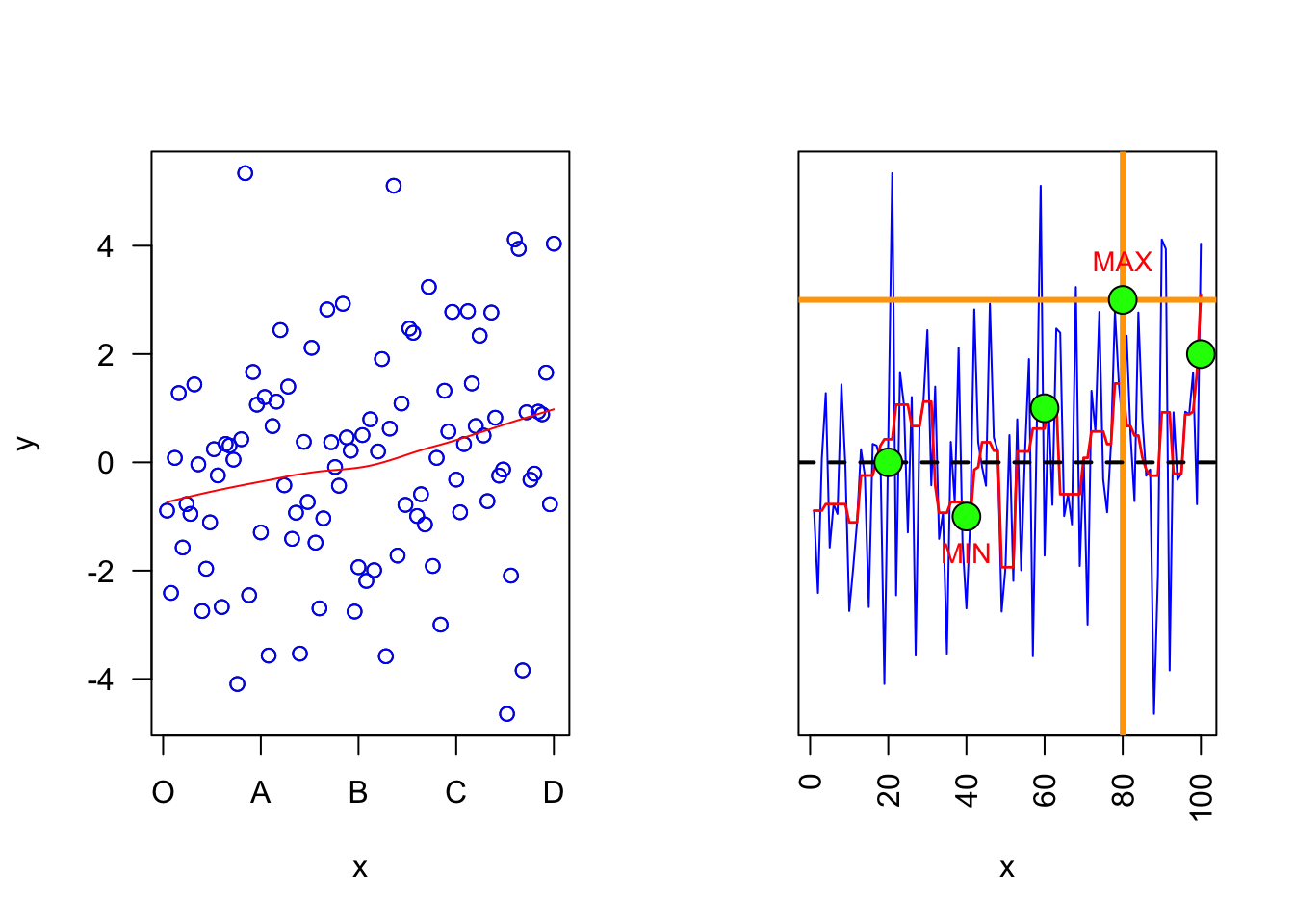## Probability density functions

par(mfrow = c(1,2))

## Left plot
plot(density(y, bw = 2), main = "")

## Right plot
y2 = rnorm(100, 4, 2)
plot(density(y, bw = 1), col = "blue", main = "")
lines(density(y2, bw = 1), col = "red")
legend("topleft", legend = c("Line 1", "Line 2"), title = "Line types", lty = 1:2, lwd = 1,  col = c("red", "blue"), cex = 0.7, box.lty = 0, bg = rgb(0,0,0,0))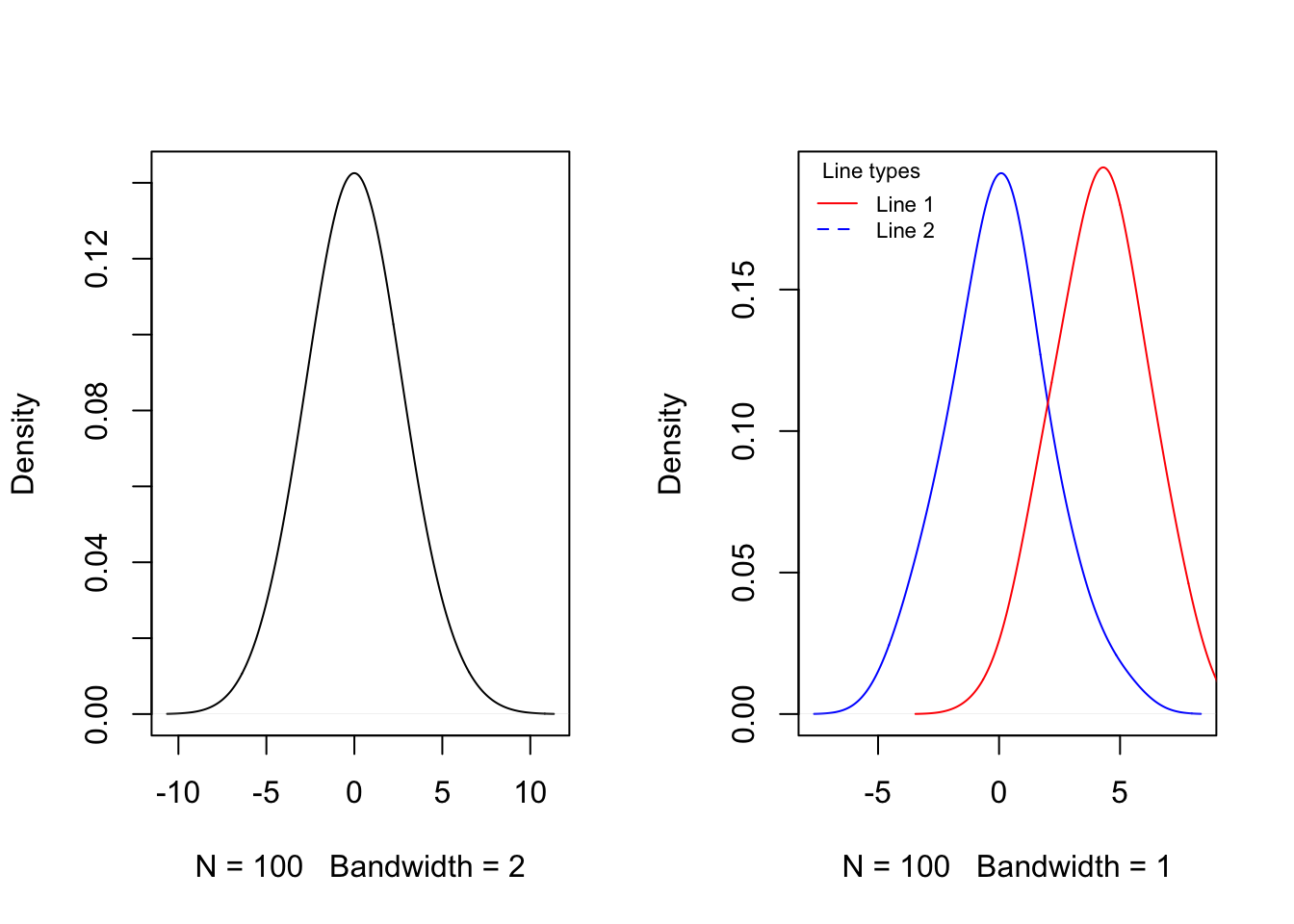## Left plot
seq = seq(-10, 10, length.out = 100)
f = dnorm(seq, 0, 2)
plot(seq, f, col = colorRampPalette(c("red","yellow","springgreen","royalblue"))(100))
legend("topright", legend = c("Point 1", "Point 2"), title = "Point types", pch = c(22,21),  col = c("red", "blue"), pt.bg = c("gold", "green"), cex = 0.75, pt.cex = 1.2, box.col = "black", box.lty = 1 , box.lwd = 1, inset = c(0.02, 0.015))
polygon(seq, f, density = 10, col = "gray", border = NA)

## Right plot
plot(seq, f, type = "l", col = "red", lwd = 3)
polygon(seq, f, density = NA, col = "gold", border = NA)
polygon(c(2, seq[seq >= 2]), c(0, f[seq >= 2]), density = NA, col="green", border = NA)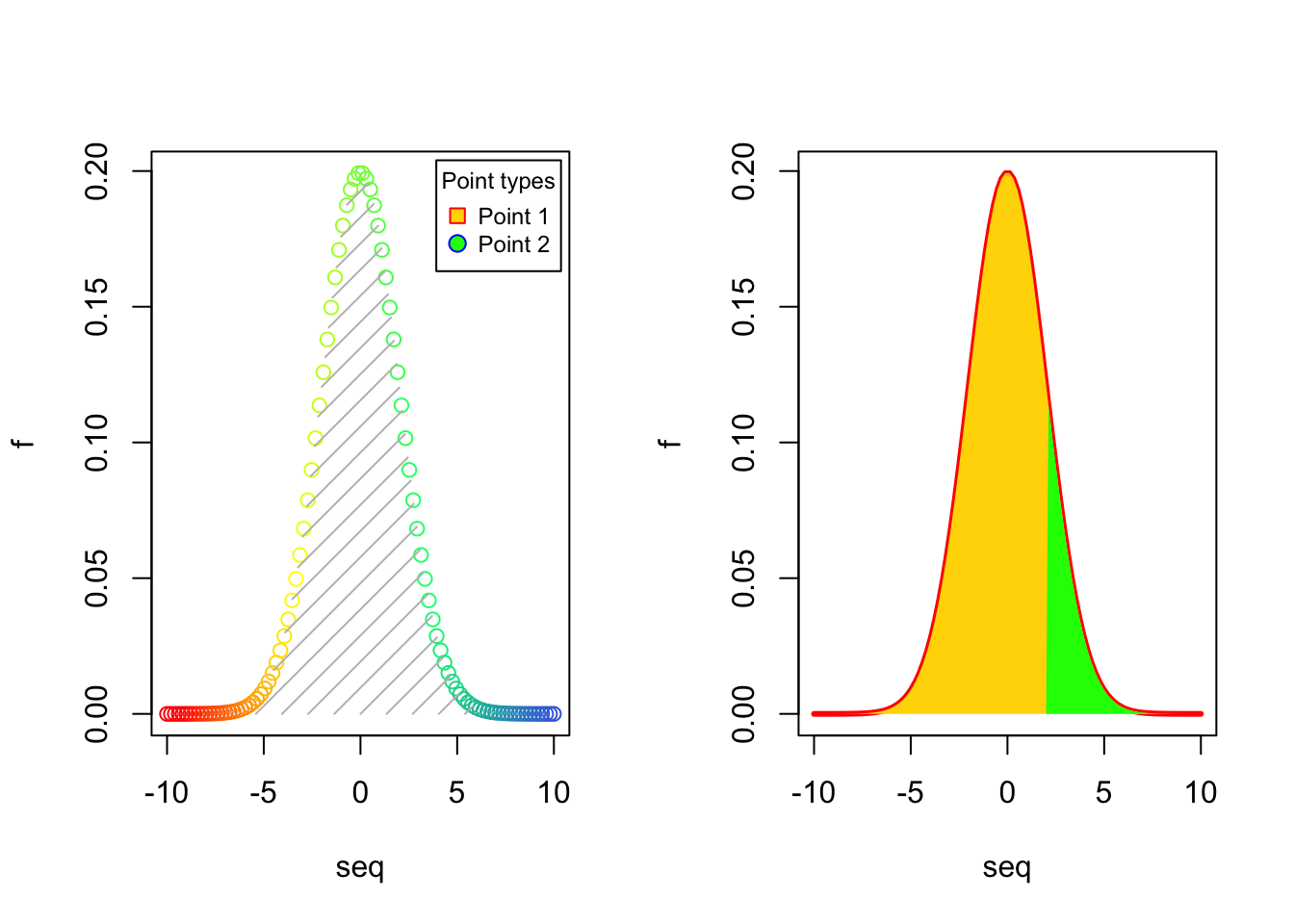## Histograms

par(mfrow = c(1,2))

## Left plot
hist(y, col = "orange", density = 10, main = "", xlab = "")

## Right plot
hist(y, col = cm.colors(10), probability = TRUE, main = "", xlab = "")
lines(seq, f, col = "blue", lty = 1, lwd = 2)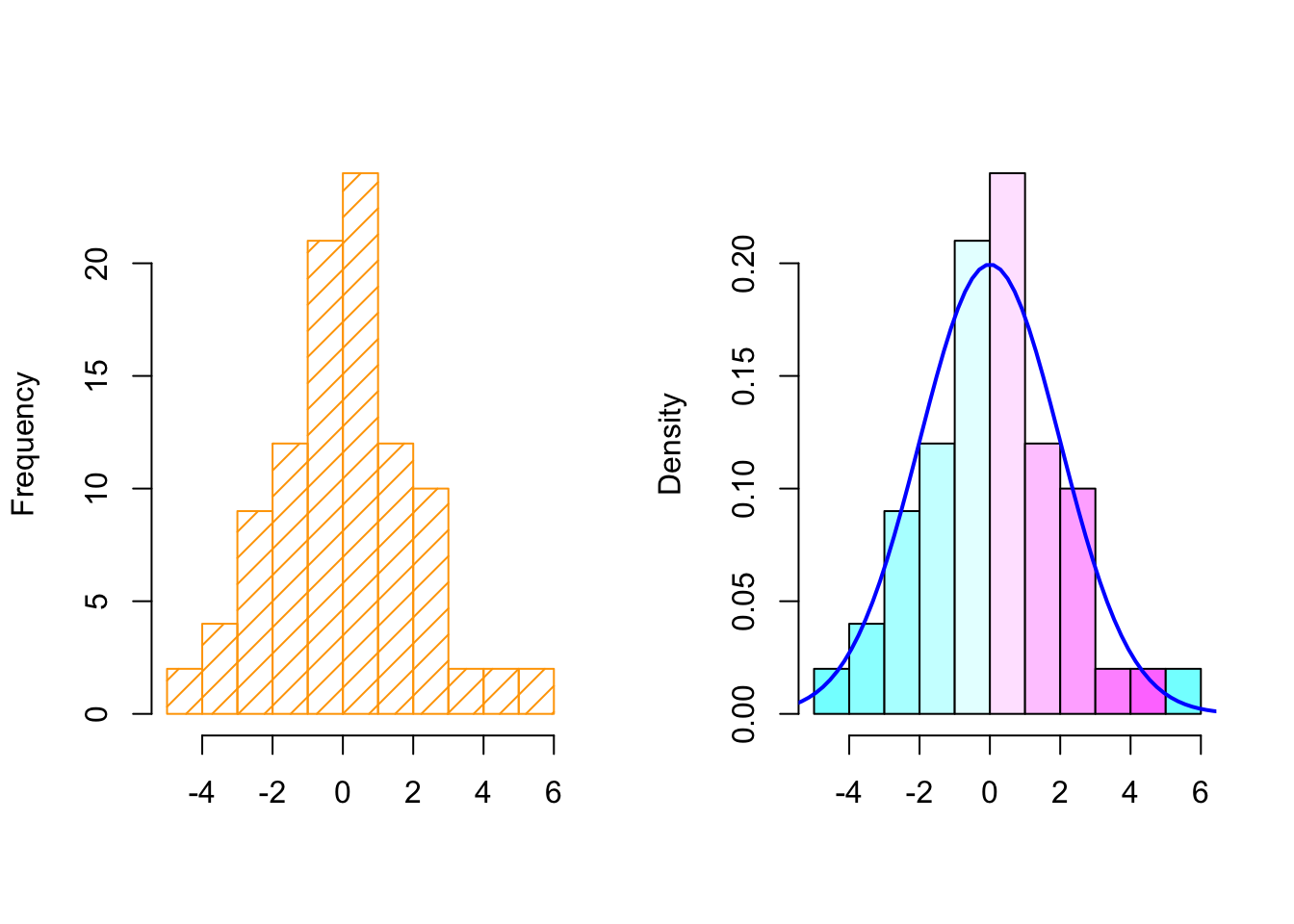## Box plots and autocorrelations

par(mfrow = c(1,2))

## Left plot
boxplot(y, y2, col = c("orange", "gold"))
abline(h = 0)

## Right plot
acf(y, main = "")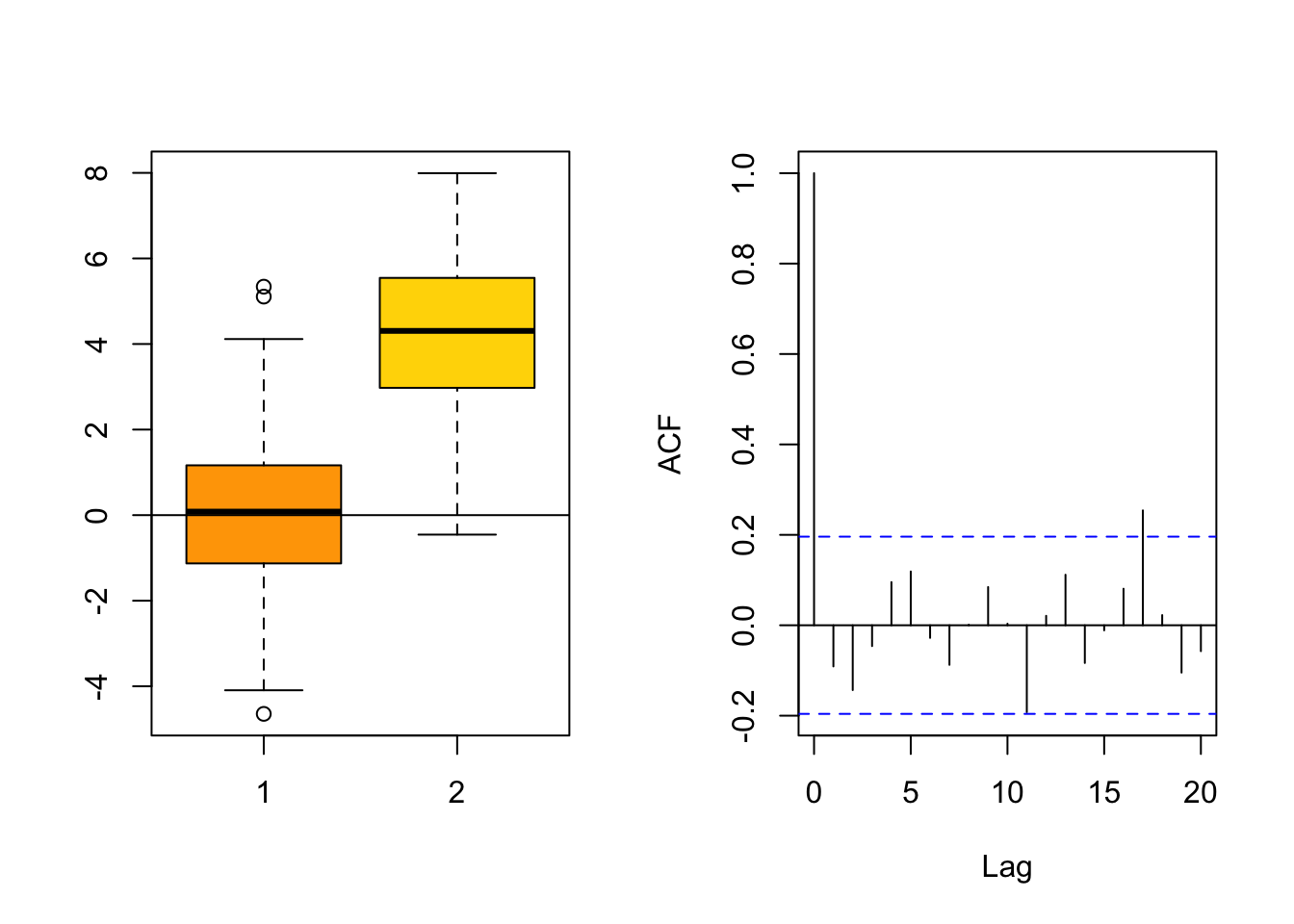boxplot(count ~ spray, data = InsectSprays, ylab = "Number of var", col = cm.colors(6))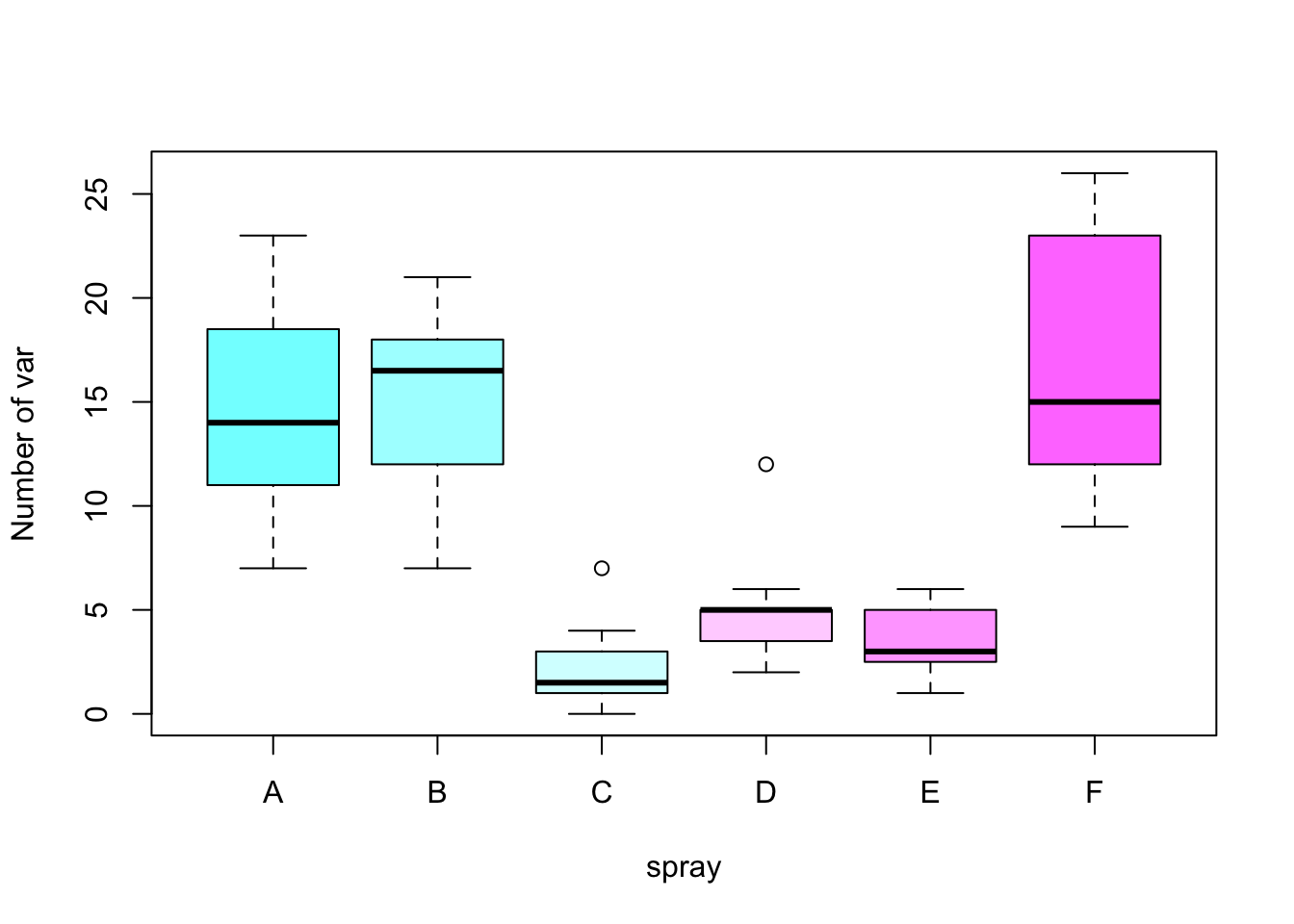## Barplots and dotcharts

par(mar = c(5,4,4,6), xpd = TRUE)
barplot(VADeaths, border = "dark blue", col = rainbow(20), cex.axis =  1.3)
legend("topright", legend = c("Group 1", "Group 2", "Group 3", "Group 4", "Group 5"),  title = "Group types", fill = c(rainbow(20)), cex = 0.8,  box.lty = 0, inset = c(-0.2,0))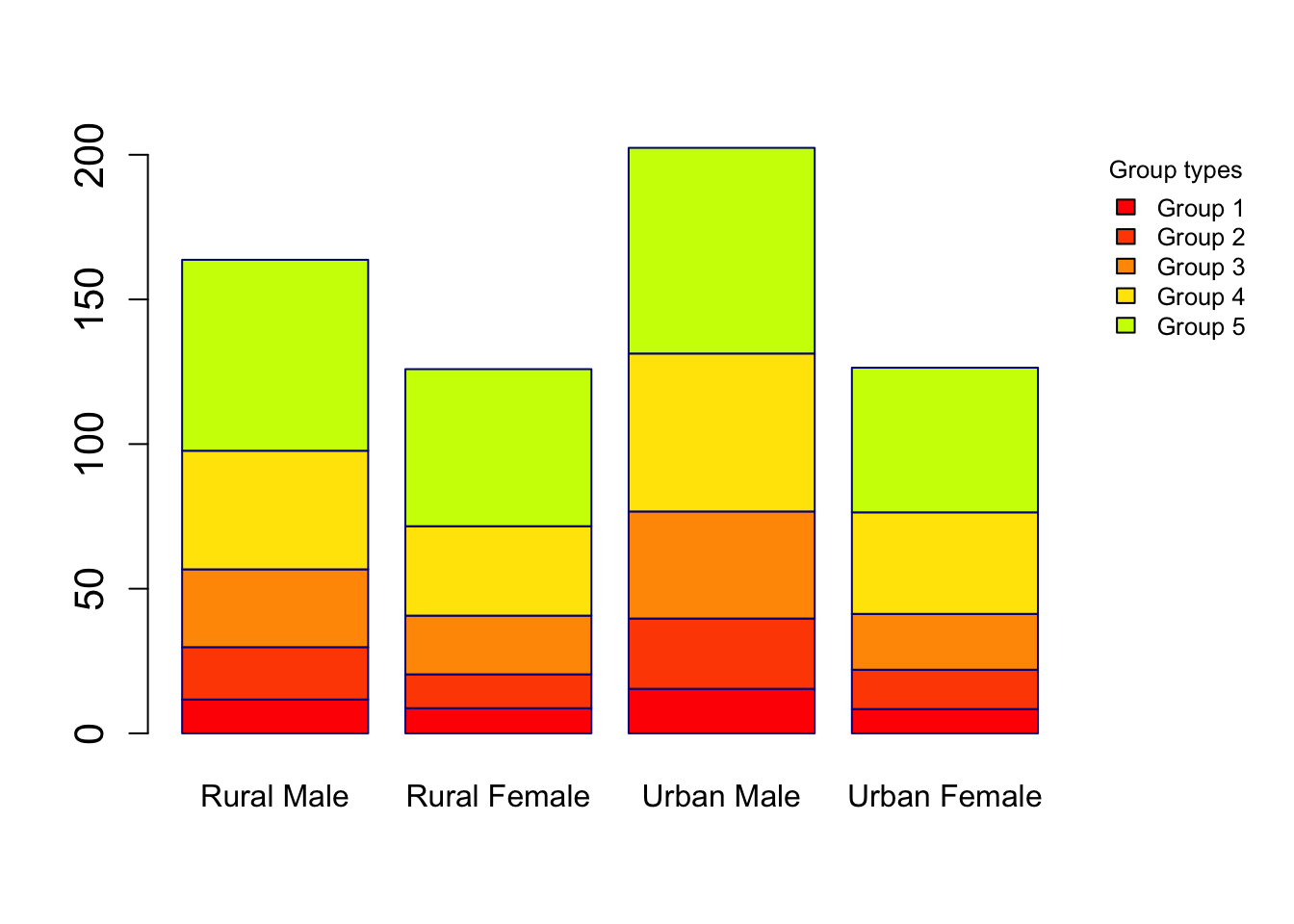eu_data = data.frame(c(1.75,4.5), c(2.8,6.3), c(3.9,6.8), c(4.35,7.2), c(5,8.25),  c(5.3,8.8))
colnames(eu_data) = 2012:2017
rownames(eu_data) = c("US","World")

barplot(as.matrix(eu_data), beside = TRUE, ylab = "Million metric tons", ylim = c(0,10), las = 1, col = gray.colors(2))
legend("top", c("US","World"), fill = gray.colors(2), horiz = TRUE, bty = "n")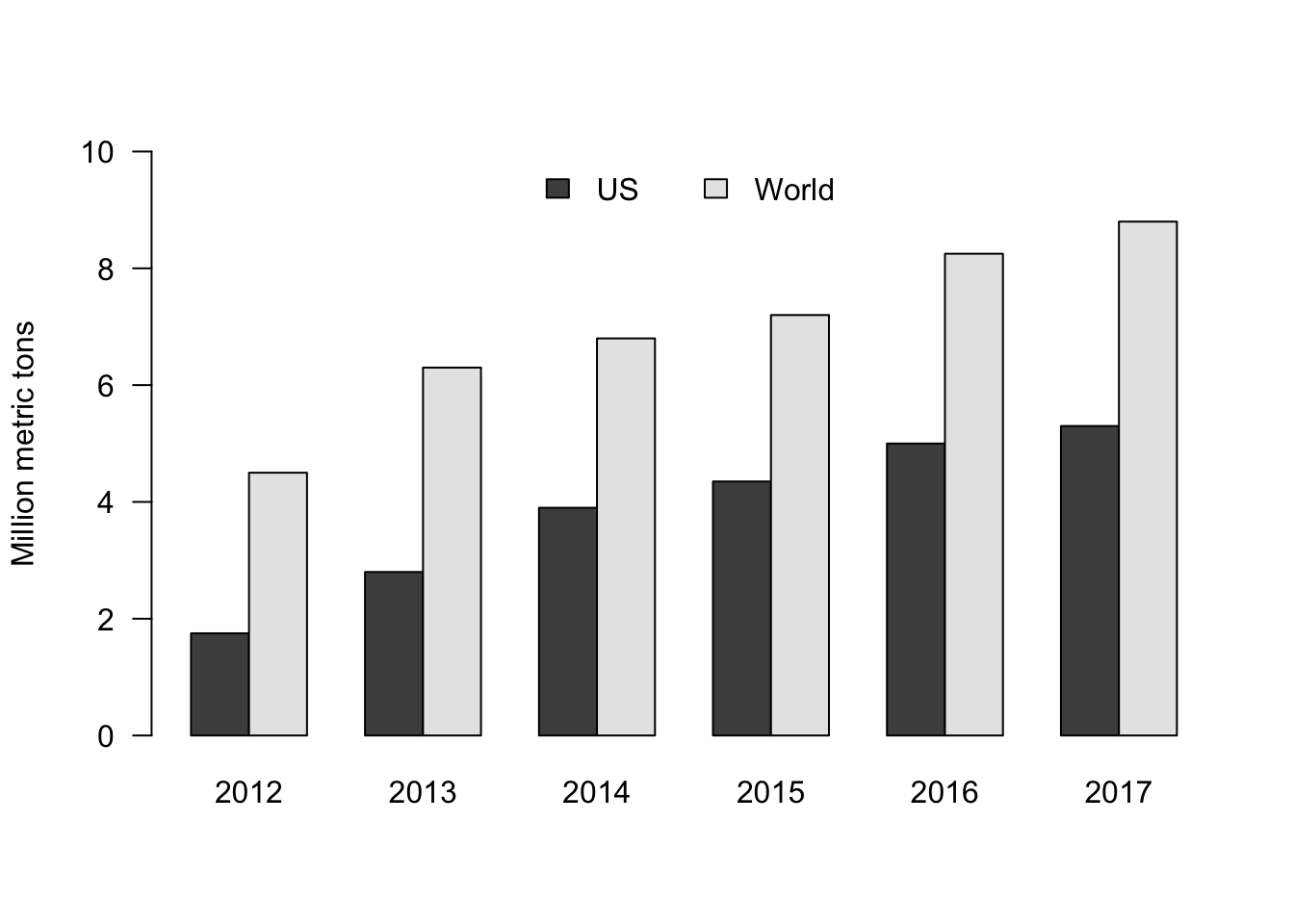dotchart(VADeaths, main = "Death Rates in Virginia1940")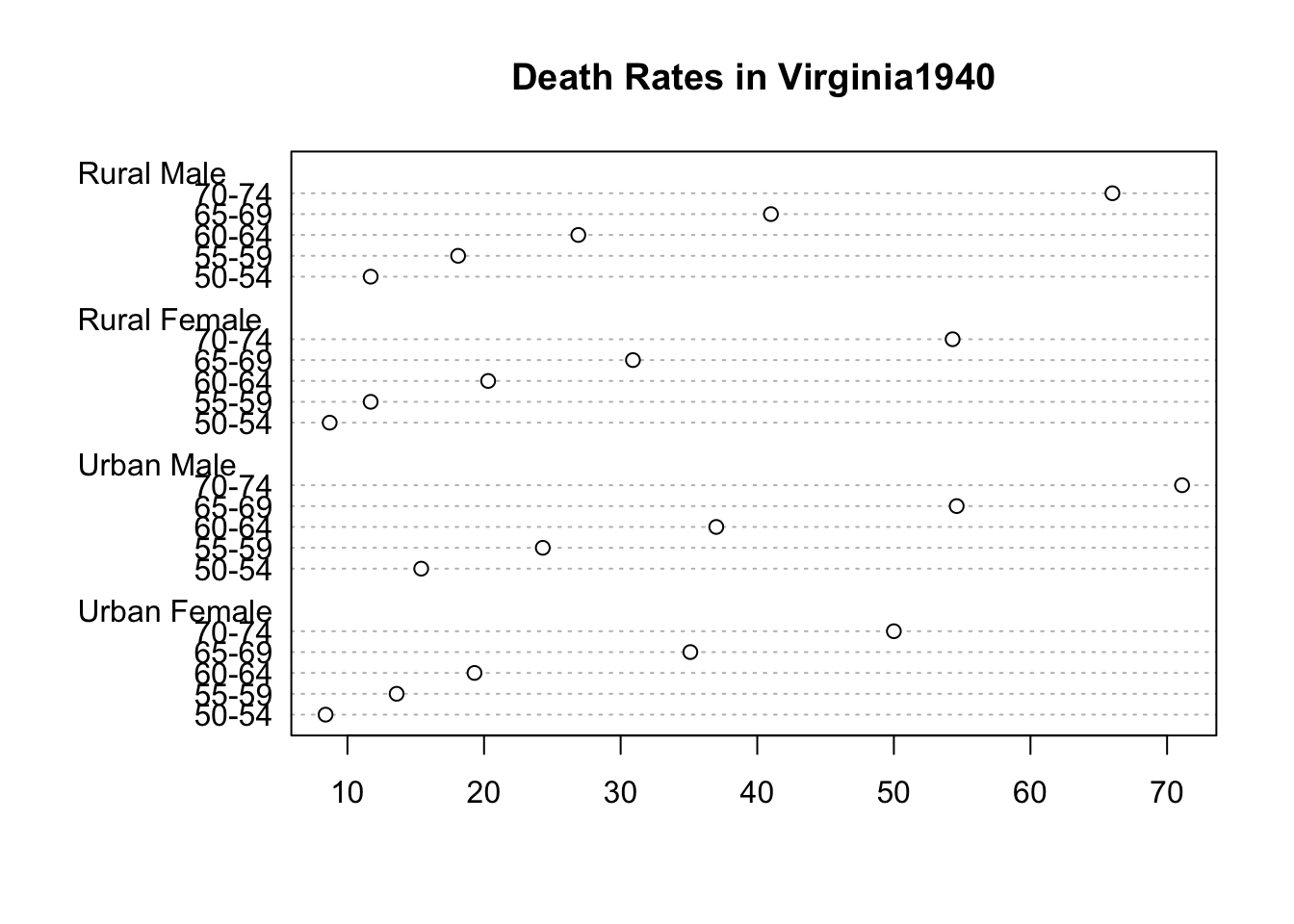## Matrix of scatter plots

pairs(iris[1:4], lower.panel = panel.smooth, upper.panel = NULL, cex = 0.75, cex.labels = 1.5, col = c("red", "blue", "green")[iris$Species], main = "Iris Data - 3 species")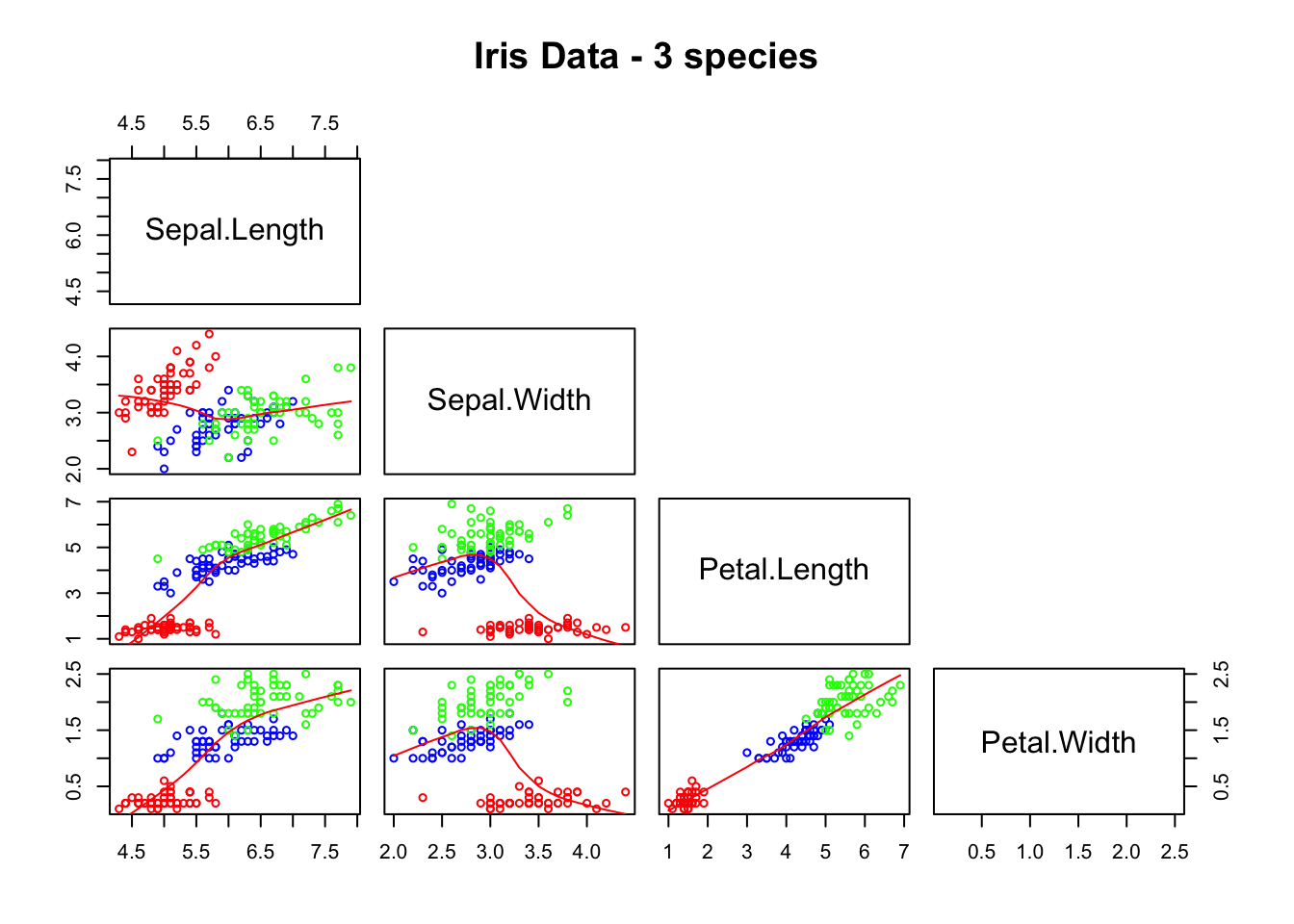pairs(~Sepal.Length + Petal.Length + Species, data = iris, subset = iris$Sepal.Length < 7 , main = "Sepal Length < 7", col = rainbow(length(levels(iris$Species)))[iris$Species])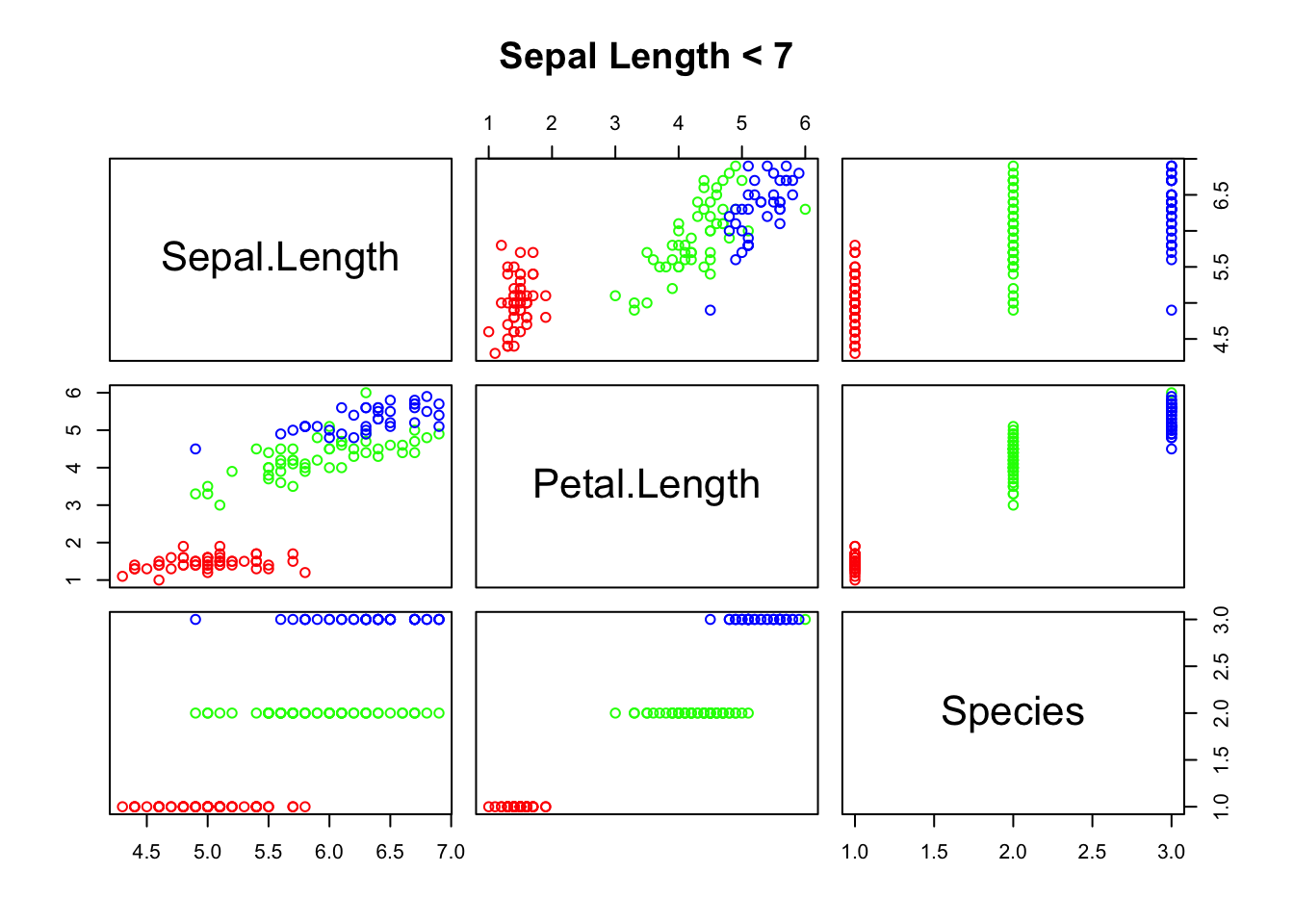## Hierarchical clustering

hca = hclust(eurodist)
plot(hca, main = "Distance between European Cities", hang = -1, ylab = "")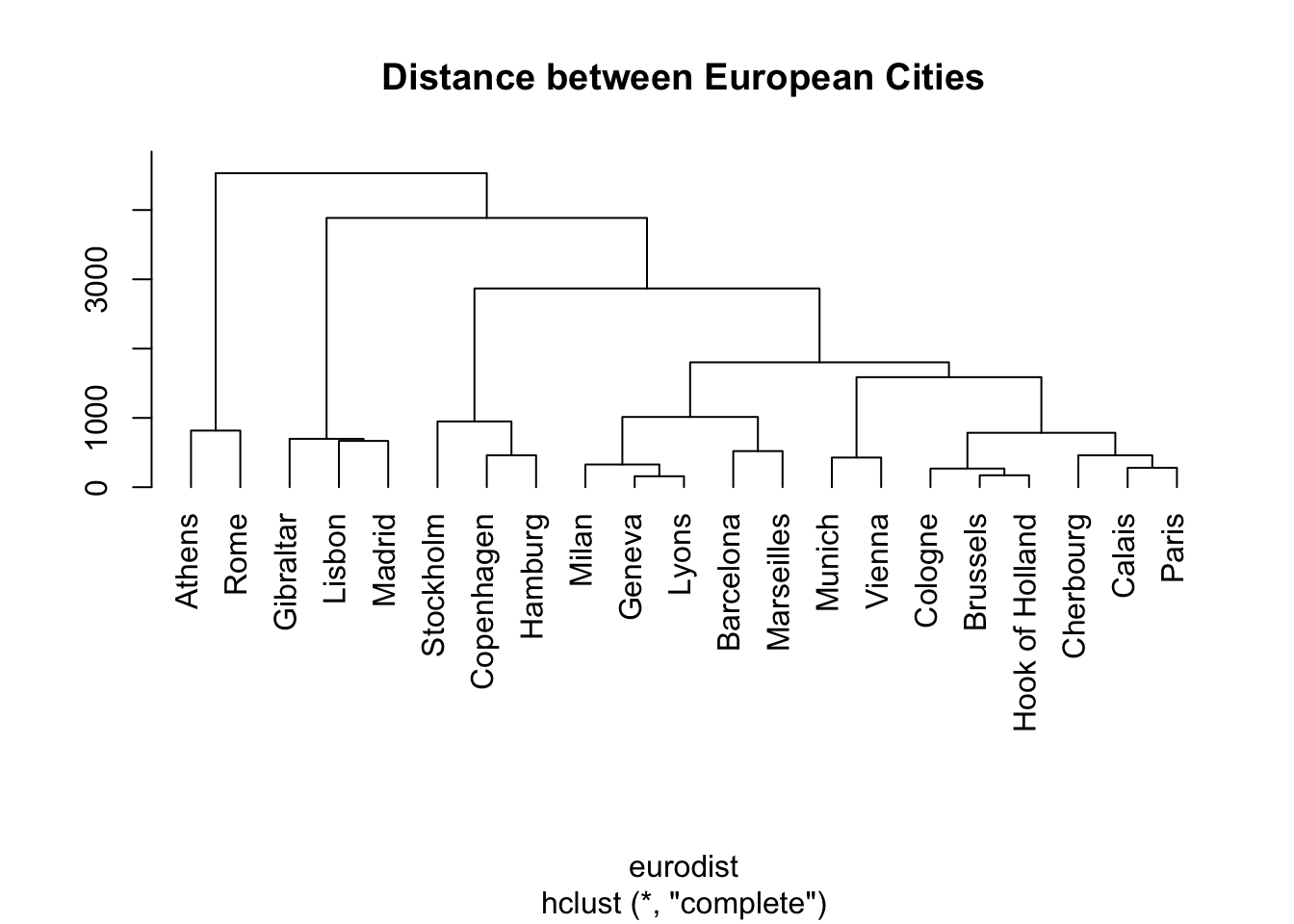## Time series

ts_data = ts(matrix(rt(300*2, df = 3), 300,2), start = c(1961, 1), frequency = 12)
plot(ts_data, main = "Time series")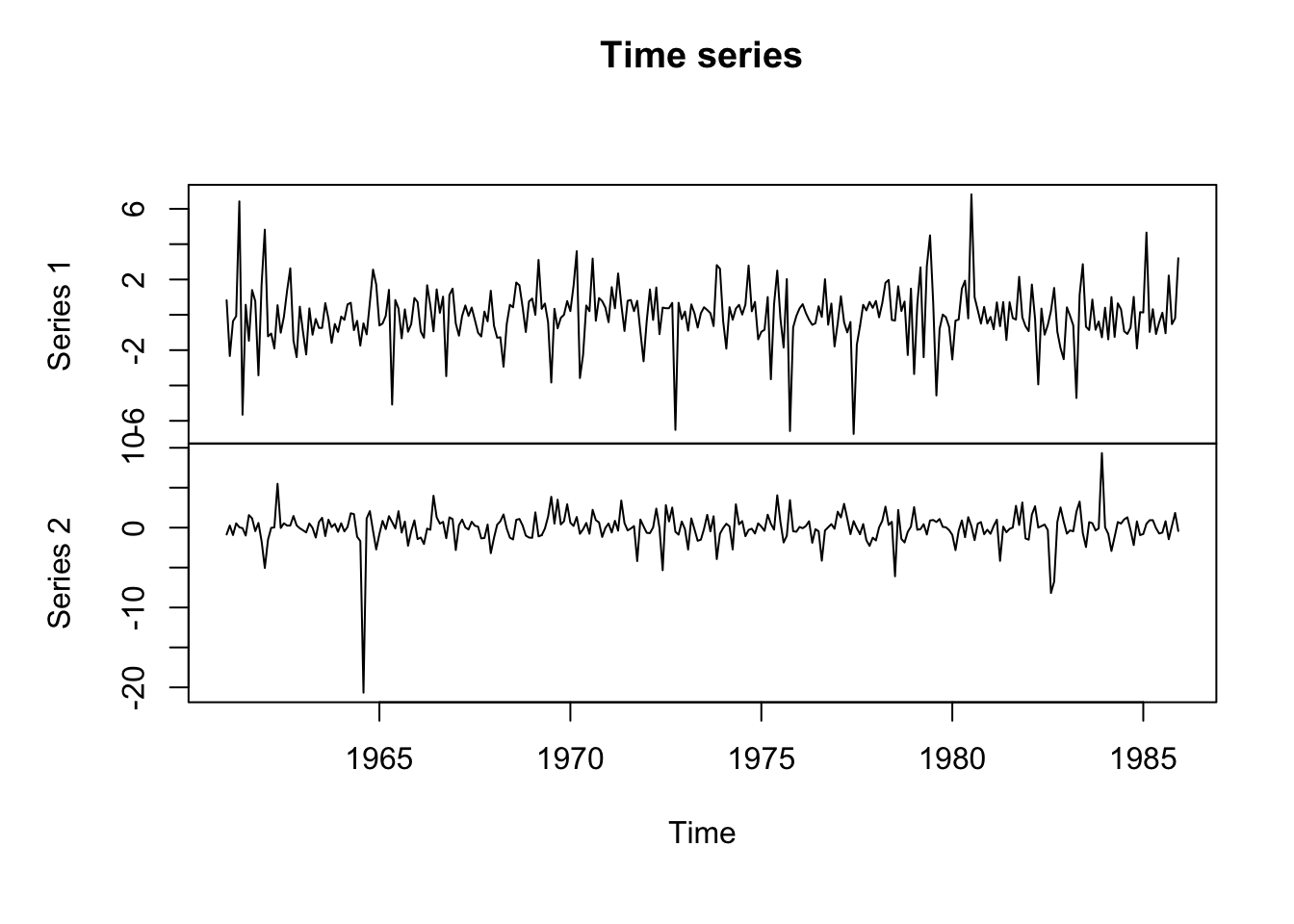## Draw functions

For instance we can draw y = 4sin(x) + (1 − cos(x)) by:

f = function(x) {
return(4 * sin(x) + (1 - cos(x)))
}

a = c()
seq_ = seq(-2*pi, 2*pi, len = 1000)
for (x in seq_) {
a = c(a,f(x))
}

plot(x = seq_, y = a, type = "l", xlab = "x", ylab = "y", col = "red")
lines(x = seq_ + 0.5, y = a, col = "blue")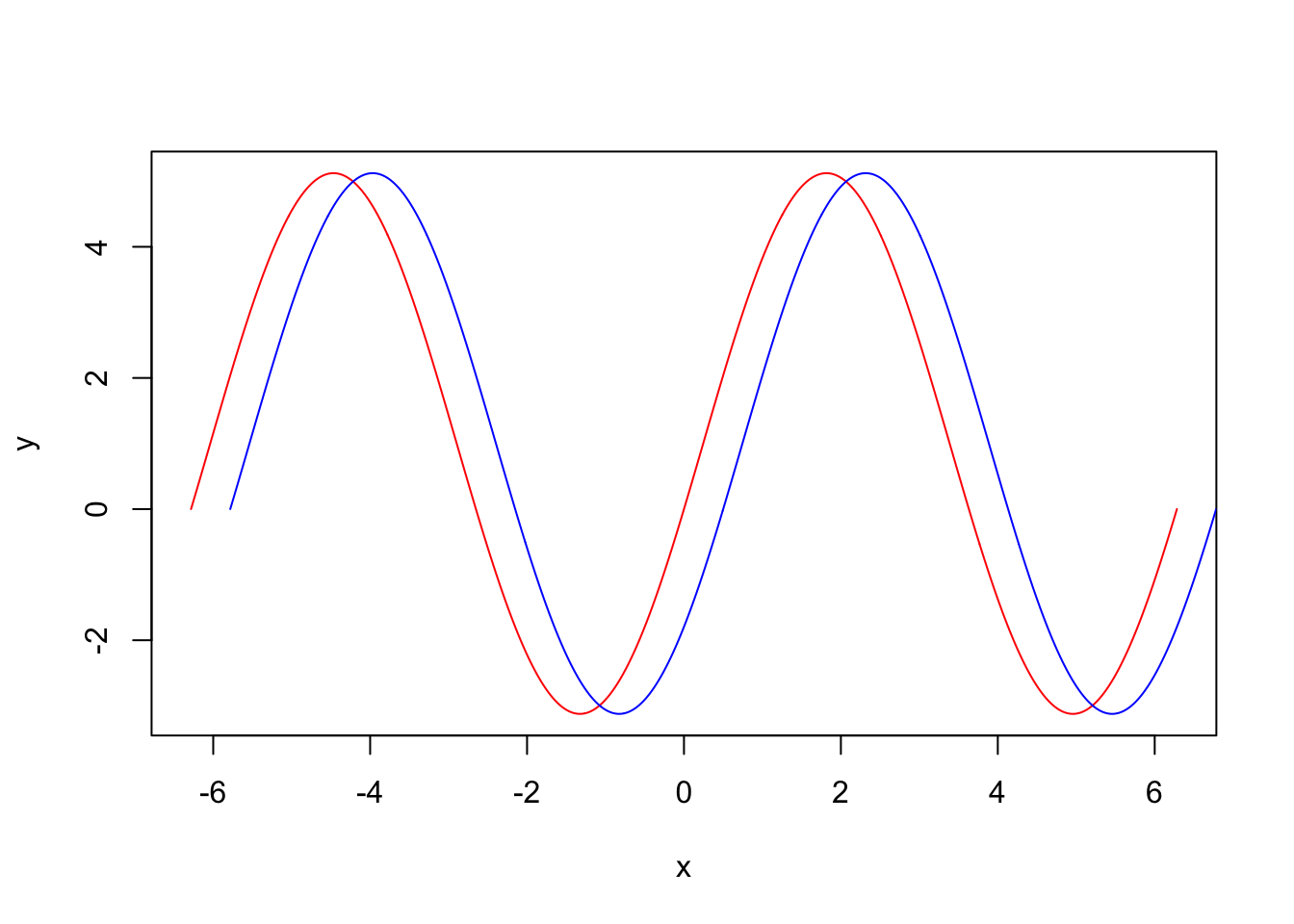For another example let’s draw a Ergodic mean plots:

par(mfrow = c(1,1))
ErgodicMean = function(x, n) {
quantile_ = matrix(0,n,3)
for (i in 1:n) {
quantile_[i,] = quantile(x[1:i], probs = c(0.5,0.025,0.975))
}
plot(quantile_[,1], ylim = c(min(quantile_),max(quantile_)), type = "l", ylab = "")
lines(quantile_[,2], lty = 2, col = "red")
lines(quantile_[,3], lty = 2, col = "blue")
}

y = rnorm(100, 0, 2)
ErgodicMean(y, 100)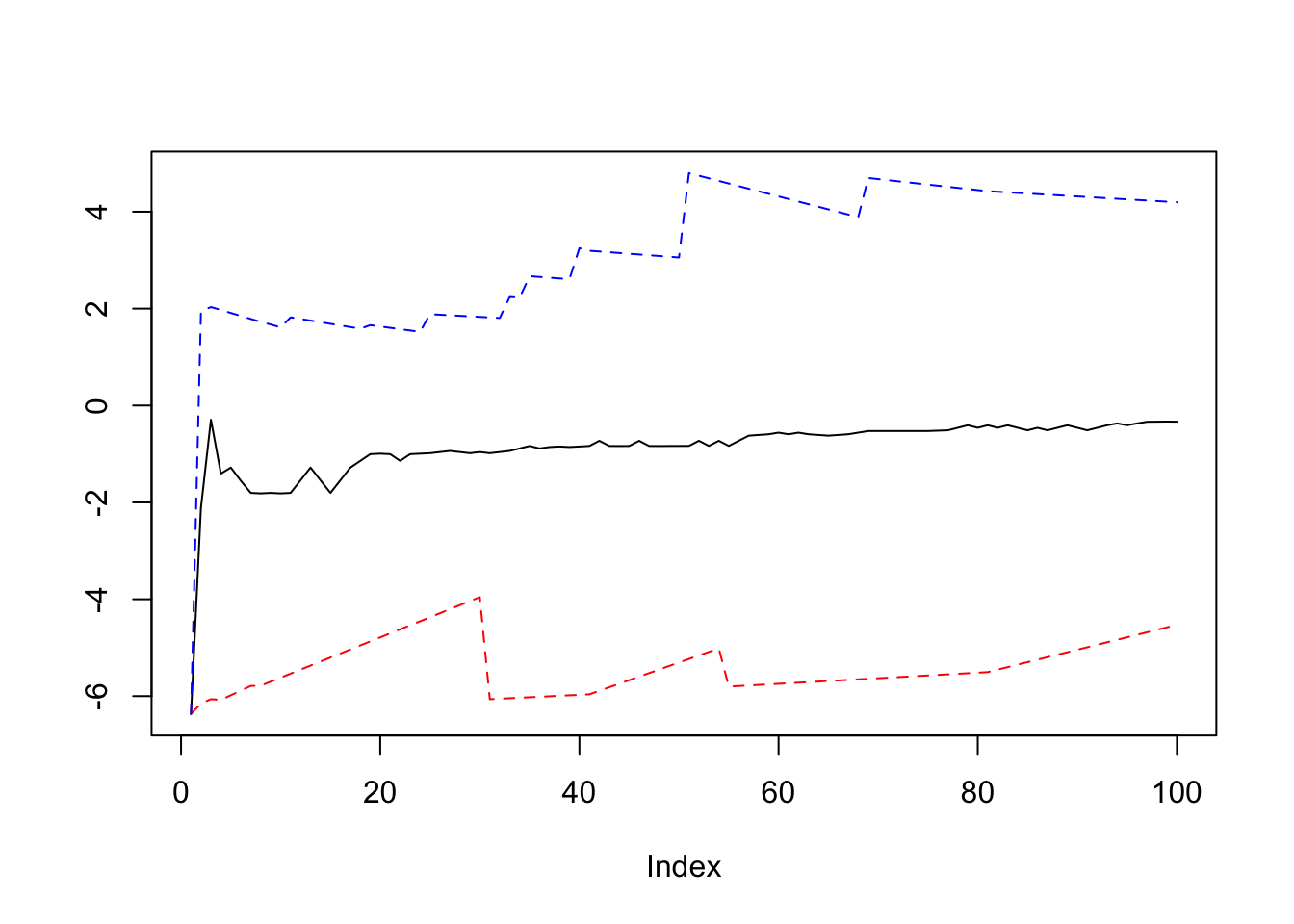## Symbols and stars

par(bty = "n", xaxt = "n", yaxt = "n", ann = FALSE)
symbols(c(0,6), c(2,8), circles = c(2,2), inches = FALSE, bg = c("green", "green"), xlim = c(-2,8), ylim = c(0,10), asp = 1)
symbols(c(0,6), c(8,2), squares = c(4,4), inches = FALSE, bg = c("blue", "blue"), add = TRUE)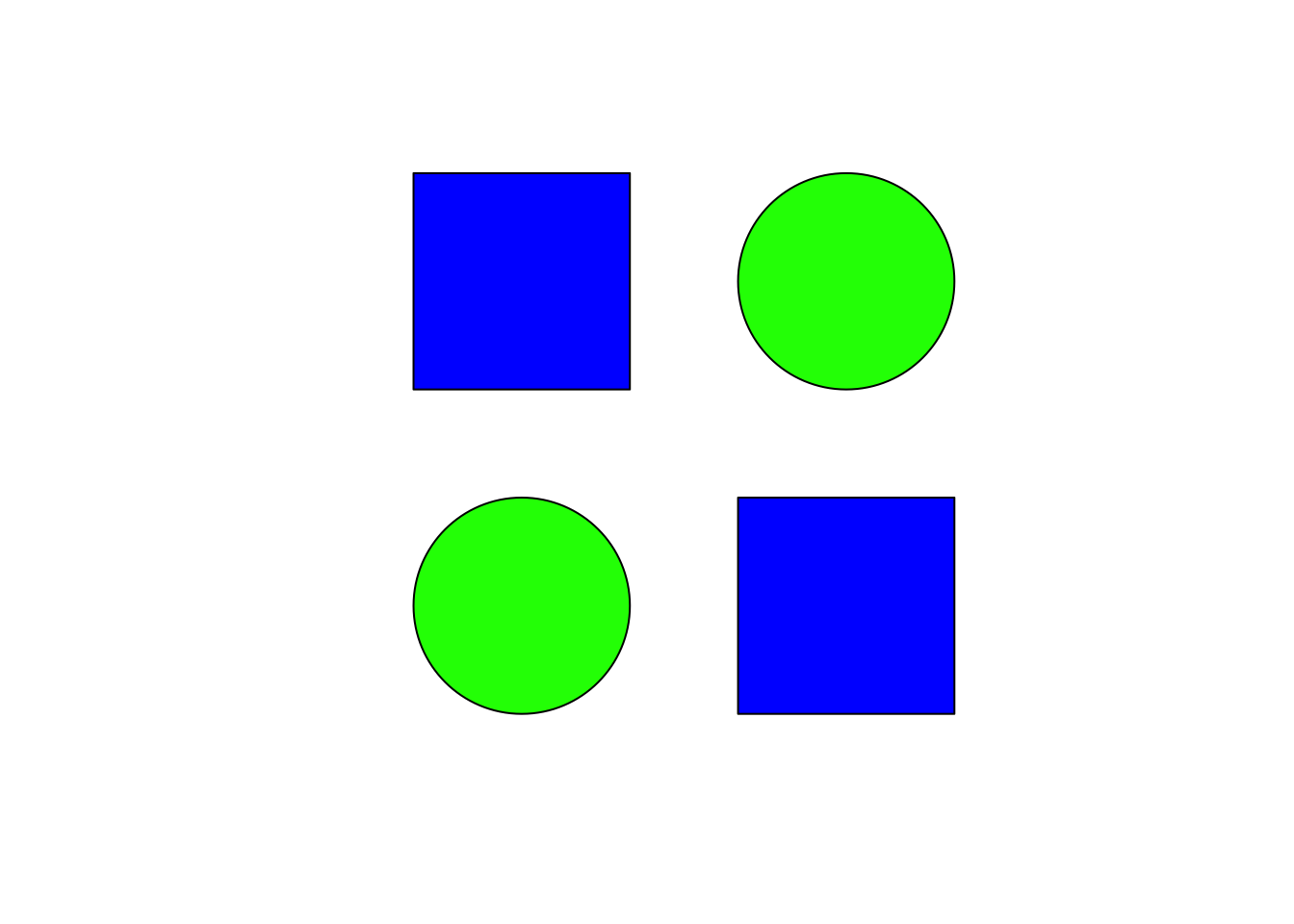stars(mtcars[, 1:7], len = 0.8, key.loc = c(12, 1.5), main = "Motor Trend Cars", draw.segments = TRUE)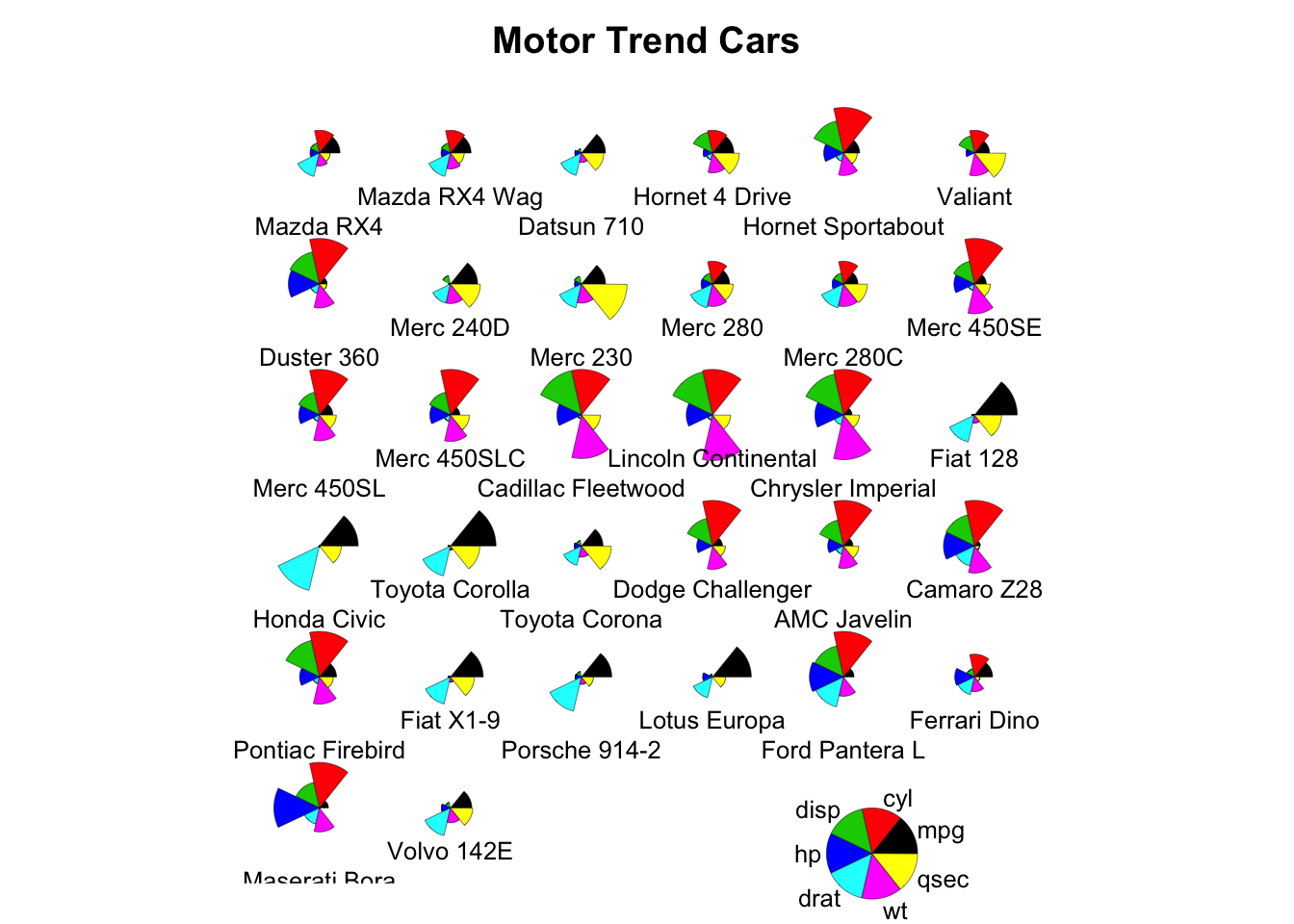## Contour, image and perspective

## Persian Rug Art (R user manual example)
x = y = seq(-4*pi, 4*pi, len = 27)
r = sqrt(outer(x^2, y^2, "+"))
par(mfrow = c(2,2), mar = rep(0, 4), xpd = FALSE)
for(f in pi^(0:3)){
contour(cos(r^2)*exp(-r/f), drawlabels = FALSE, axes = FALSE, frame = TRUE)
}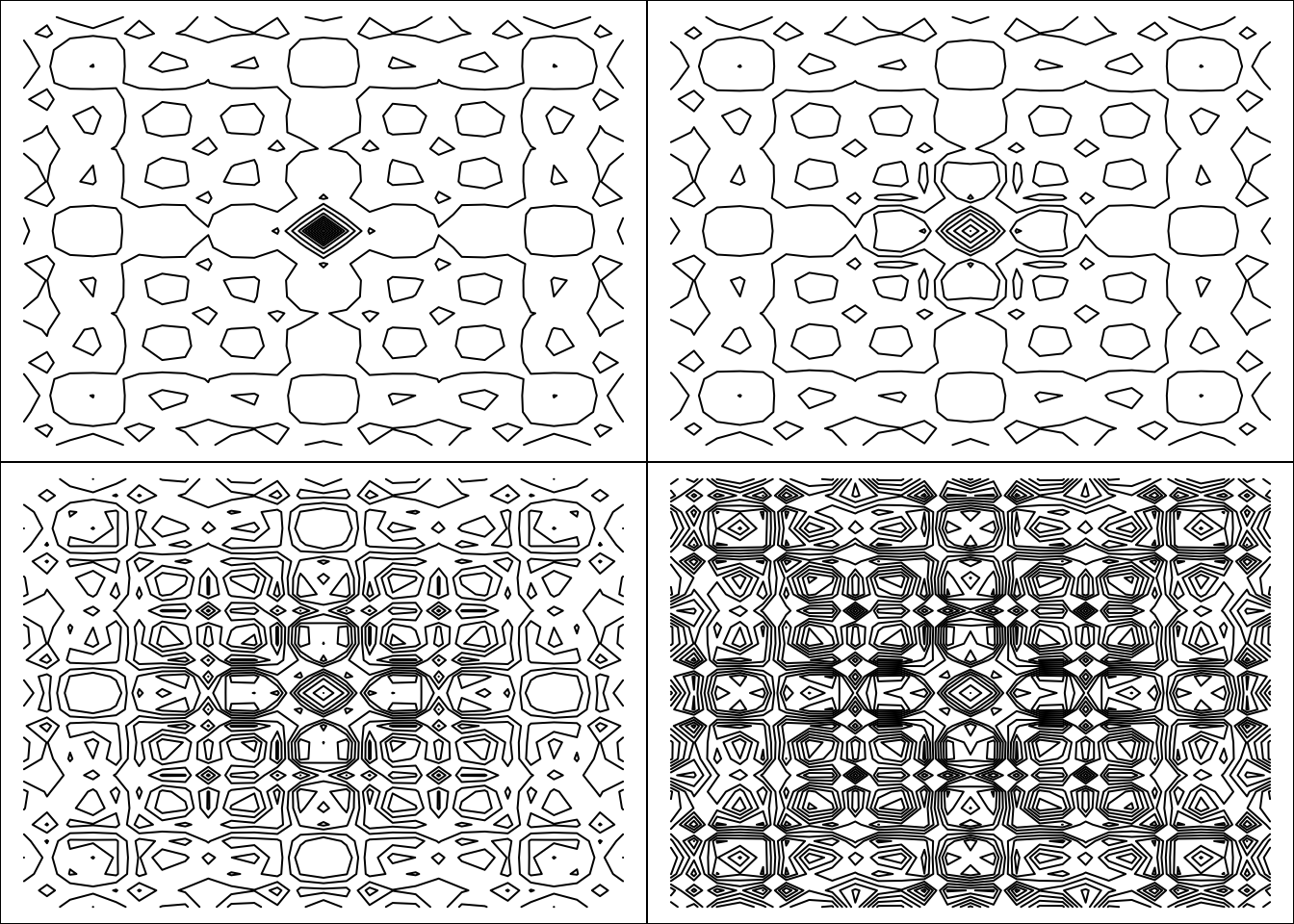x = y = -6:16
z = outer(x, sqrt(abs(x)), FUN = "/")
image(x, y, z)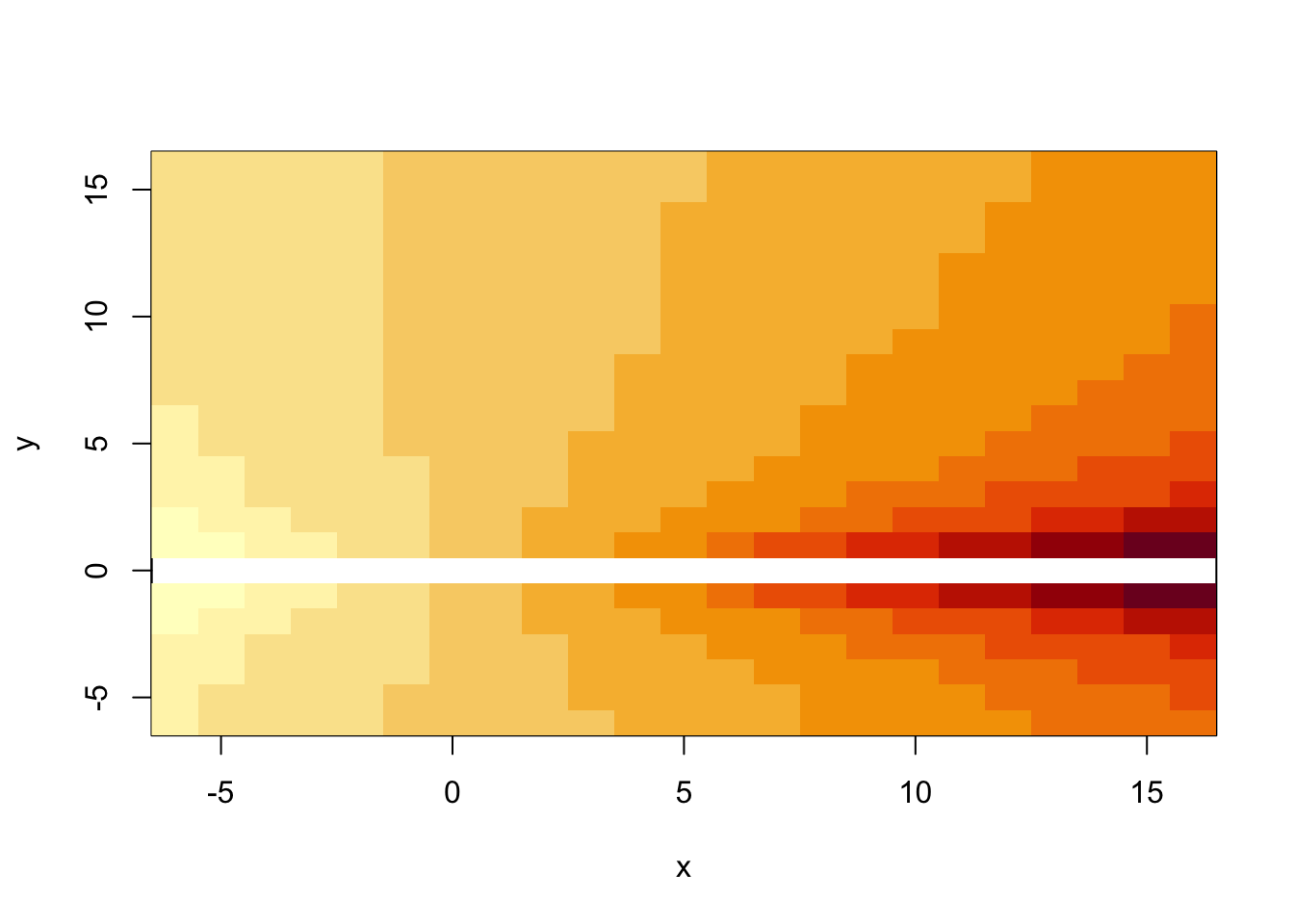x = y = seq(-10, 10, length = 30)
f = function(x, y) {r = sqrt(x^2 + y^2); 10 * sin(r)/r}
z = outer(x, y, f)
z[is.na(z)] = 1
persp(x, y, z, theta = 30, phi = 30, expand = 0.5, col = "lightblue")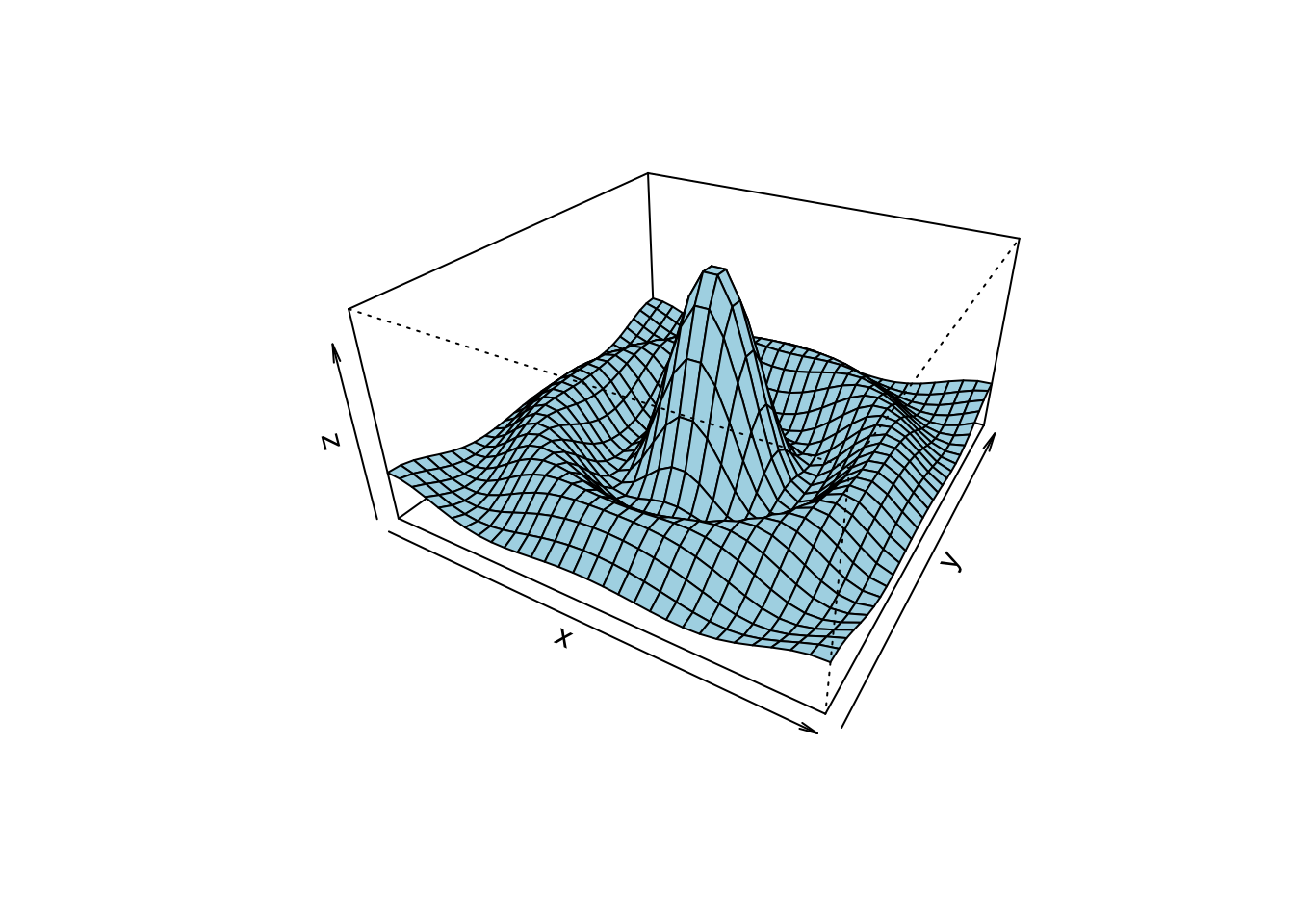## Tables

In R we can use kable function from knitr library to print tables in HTML or LaTeX formats. kable is a great tool, but the generated outputs are usually not formatted for including in reports. While building Markdown or Latex reports it will be useful to use the following packages to make them more publication quality:

For example:

library(knitr)
library(kableExtra)
cardata = mtcars
kable(head(cardata), format = "html", caption = "Title of the table") %>%
kable_styling(full_width = FALSE, font_size = 16, position = "center") # position: left, center, right, float_left and float_right
mpg cyl disp hp drat wt qsec vs am gear carb
Mazda RX4 21.0 6 160 110 3.90 2.620 16.46 0 1 4 4
Mazda RX4 Wag 21.0 6 160 110 3.90 2.875 17.02 0 1 4 4
Datsun 710 22.8 4 108 93 3.85 2.320 18.61 1 1 4 1
Hornet 4 Drive 21.4 6 258 110 3.08 3.215 19.44 1 0 3 1
Hornet Sportabout 18.7 8 360 175 3.15 3.440 17.02 0 0 3 2
Valiant 18.1 6 225 105 2.76 3.460 20.22 1 0 3 1
fm = lm(mpg ~ cyl + disp + hp, data = cardata)
sm = round(summary(fm)$coef,3) # Adding some cosmetic to columns that we want row.names(sm) = cell_spec(row.names(sm), "html", bold = TRUE) sm[,4] = cell_spec(sm[,4], "html", color = ifelse(sm[,4] < 0.1, "red", "black")) kable(sm, "html", escape = F) %>% kable_styling(bootstrap_options = "hover", full_width = FALSE, font_size = 16, position = "center") Estimate Std. Error t value Pr(>|t|) (Intercept) 34.185 2.591 13.195 0 cyl -1.227 0.797 -1.54 0.135 disp -0.019 0.01 -1.811 0.081 hp -0.015 0.015 -1.002 0.325 ## Maps R does not have default functions for drawing maps and shapefiles. To generate maps, we can use the following packages: • maps: draw lines and polygons as specified by a map database • sf: provide Simple Features for R. Find more details about sf in here and in the cheetsheet • leaflet: create interactive maps. The widget can be rendered on HTML pages generated from R Markdown, Shiny, or other applications. You may learn more about leaflet in here ## Layout layout creates a layout for putting multiple plots together. Number of rows and columns are specified in a matrix, with the column-widths and the row-heights specified in the respective arguments. lay = layout(matrix(c(1,0,2,3,4,0),3,2,byrow = TRUE), widths = c(4,1.5), heights = c(1.5,4,1), respect = TRUE) layout.show(lay)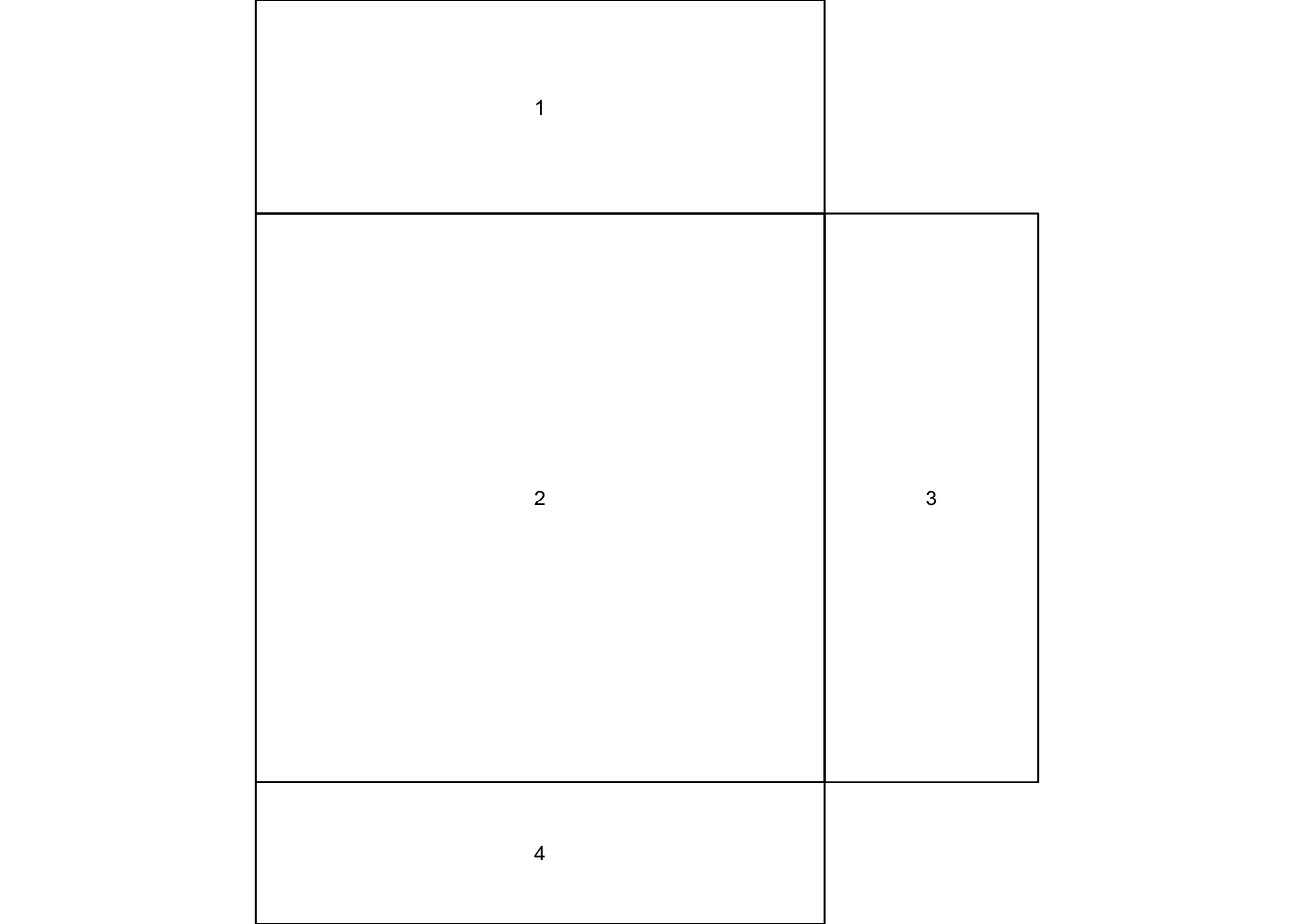x = pmin(3, pmax(-3, rnorm(100))) y = pmin(3, pmax(-3, rnorm(100))) xhist = hist(x, breaks = seq(-3,3,0.5), plot = FALSE) yhist = hist(y, breaks = seq(-3,3,0.5), plot = FALSE) top = max(c(xhist$counts, yhist$counts)) par(mar = c(0,3,3,1)) plot(xhist, col = "gray", axes = FALSE, xlab = "", ylab = "", main = "") title(main = "Main Title", cex.main = 1.5) par(mar = c(4,3,1,1)) plot(x[1:15], y[1:15], xlim = c(-3, 3), ylim = c(-3, 3), xlab = "x variable", ylab = "", cex.lab = 1.2) arrows(x[1:15], y[1:15]-0.5, x[1:15], y[1:15]+0.5, col = 4, angle = 90, length = 0.05, code = 3) par(mar = c(3,0,1,1)) barplot(yhist$counts, axes = FALSE, xlim = c(0, top), space = 0, horiz = TRUE, col = "black", bg = "gray", density = 20)

par(mar = c(0,3,0,0))
plot.new()
legend("center", legend = c("Top", "Middle", "Right"), fill = c("gray", "white", "black"), density = c(-1,-1,40), bg = "gray90", horiz = TRUE)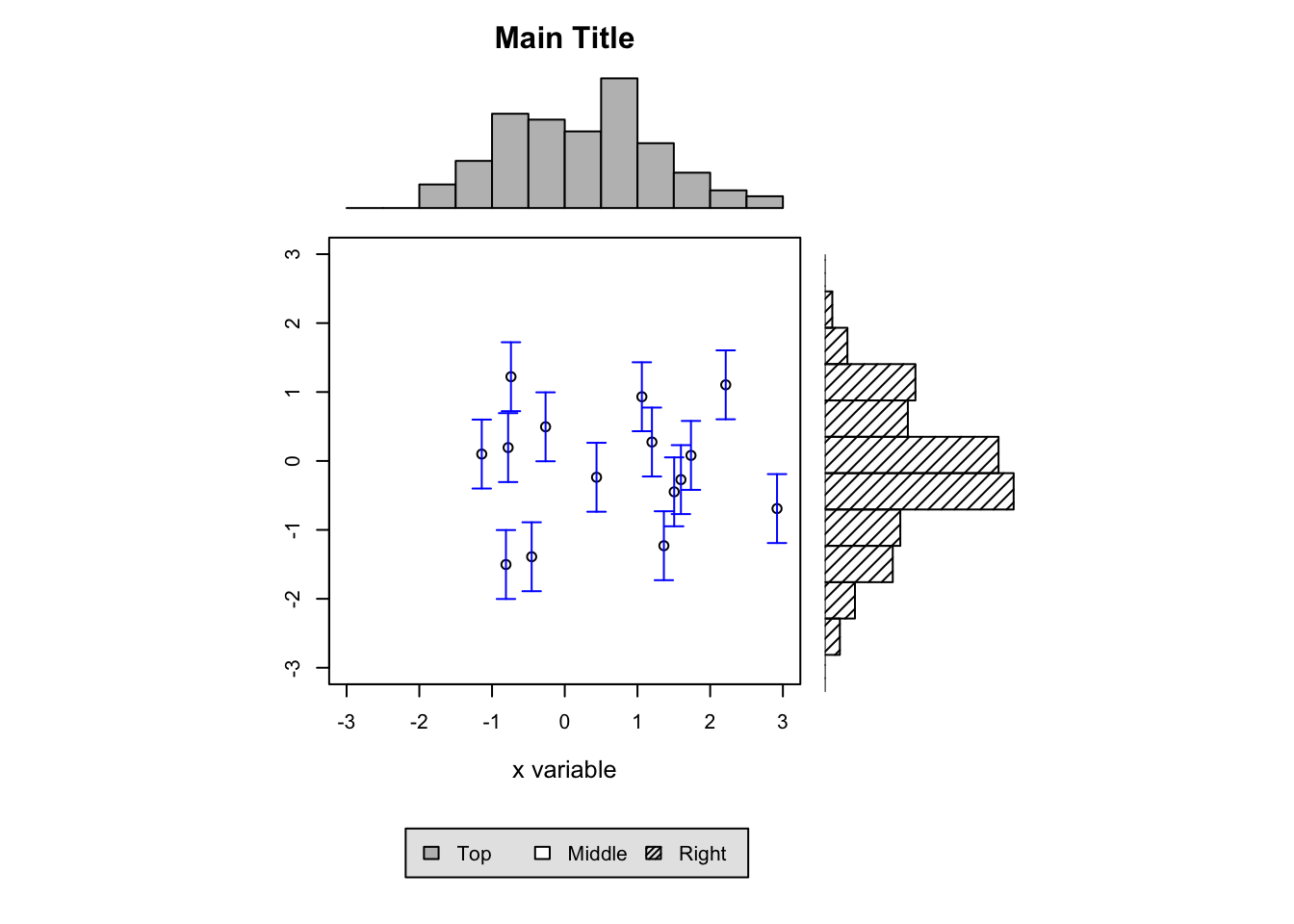## Color palettes

R also offers the following built in color palettes that help to generate color vectors of desired length quickly.

• rainbow()
• colorRampPalette()
• heat.colors()
• gray.colors()
• terrain.colors()
• topo.colors()
• cm.colors()
par(mfrow = c(1,2))
pie(rep(1, 24), col = rainbow(24), radius = 1)
pie(rep(1, 24), col = colorRampPalette(c("red","yellow","springgreen","royalblue"))(24), radius = 1)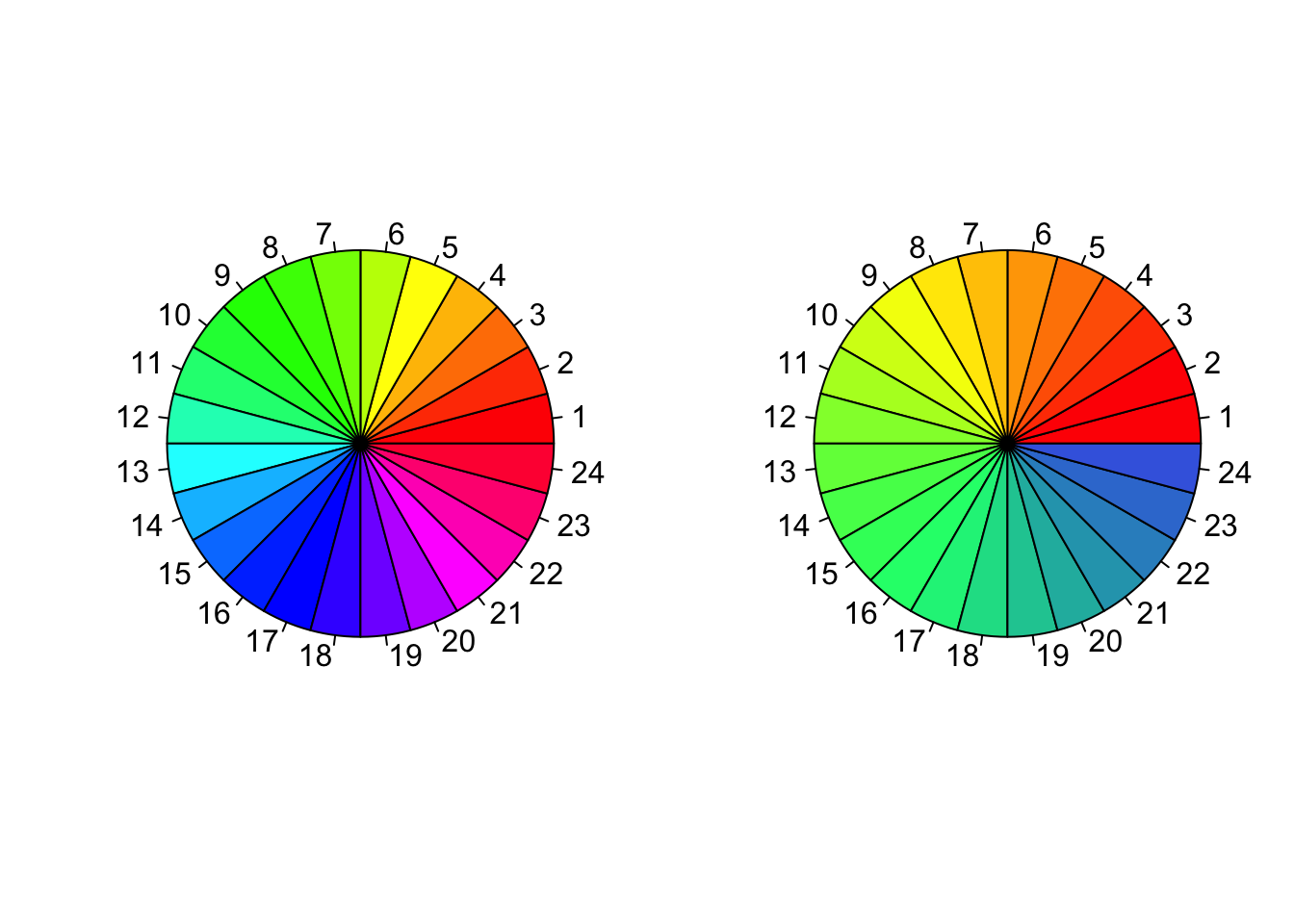pie(rep(1, 24), col = heat.colors(24), radius = 1)
pie(rep(1, 24), col = terrain.colors(24), radius = 1)pie(rep(1, 24), col = topo.colors(24), radius = 1)
pie(rep(1, 24), col = cm.colors(24), radius = 1)